Courses

# Structure Of Ionic Compounds And Defects - Solid State Chemistry Notes | EduRev

## Chemistry : Structure Of Ionic Compounds And Defects - Solid State Chemistry Notes | EduRev

The document Structure Of Ionic Compounds And Defects - Solid State Chemistry Notes | EduRev is a part of the Chemistry Course Physical Chemistry.
All you need of Chemistry at this link: Chemistry

Structure of Ionic Compounds :

Simple ionic compounds are of the type AB or AB2 where A and B represent the positively and negatively charged ions respectively. (In any solid of the type Ax, By, the ratio of co-ordination number of A to B would be y:x.

• Structures of Type AB: Ionic compounds of the type AB means compounds having the positively and negatively charged ions in the ratio 1:1. These compounds can have following three t ypes of structures.
(i) Rock salt (NaCl) type
(ii) Cesium chloride (CsCl) type
(iii) Zinc blende (ZnS) type

Structure of Ionic Compounds of the Type A2B: These are the ionic compounds having cations and anions in the ratio 1:2 Example: CaF2, SrF2, BaCl2, BaF2, PbF2, and CdF2

Structure of Ionic Compounds of the Type A2B: These are the ionic compounds having anions and cations in the ratio 1:2 Example: Na2O, Li2O, K2O, Rb2O and Rb2S

Some special structures

WURTZITE Structure

(a) Sulphide ions have hcp arrangement and zinc ions occupy tetrahedral voids
(b) Structure has 4:4 coordination

Imperfections or defects in solids

At absolute zero, crystals tend to have a perfectly ordered arrangement. This arrangement corresponds to state of lowest energy. As the temperature increases, the crystals start deviating from the perfectly ordered arrangement. Any deviation from the perfectly ordered arrangement constitutes a defect or imperfection. These defects are sometimes called thermodynamic defects because the number of these defects depends on the temperature. Crystals may also possess addition defects due to the presence of impurities. Many properties of crystalline solids such as electrical conductivity and mechanical strength can be explained in terms of imperfections. Imperfections not only modify the properties of solids but also give rise to new properties.

The defects which arise due to the irregularity in the arrangement of atoms or ions are called atomic imperfections. The atomic defects caused by missing or misplaced ions are called point defects. If the deviations from periodicity extend over microscopic regions of the crystal they are called lattice imperfections. Lattice imperfections may extend along lines (line defects) or planes (plane defects). Line defects are also called dislocations. Various types are as follows.

(i) Stoichiometric point defects : The compounds in which the numbers of positive and negative ions are exactly in the ratios indicated by their chemical formula are called stoichiometric compounds. The defects that do not disturb the stoichiometry (the ratio of numbers of positive and negative ions) are called stoichiometric defects. These are of following types.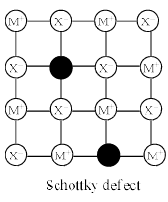(b) Interstitial Defects- This t ype of defects is caused due to the normally vacant interstitial sites in the crystal. The ions occupying the interstitial sites are called interstitials. The formation of interstitial defects is determined by the size of the interstitial ion.

(c) Frenkel Defect – This t ype of defect is created when an ion leaves its correct latt ice site and occupies an interstitial site. Frenkel defects are common in ionic compounds which have low coordination number in which there is difference in size between positive and negative ions. For exampleZnS, AgCl, AgBr and AgI.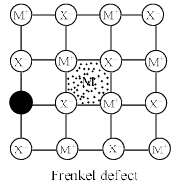Consequences of stoichiometric defects
(i) The presence of those defects in the crystal makes them good electrical conductors.
(ii) The presence of holes in the Schottky type defects lowers their density.
(iii) The presence of ions in interstitial sites in the Frenkel defects increases the dielectric constant of the crystals.

(ii) Non Stoichiometric defects

There are many compounds in which the ratio of positive and negative ions present in the compound differs from that required by ideal chemical formula of the compound. In these compounds composition may vary over a wide range. The defects which disturb the stoichiometry of the compound are called

non-stoichiometric defects. These defects are either due to the presence of excess metal ions or excess non-metal ions.

(a) Metal excess Defects due to Anion Vacancies : A compound may have excess metal ion if a negative ion is absent from its lattice site, leaving ‘hole’ which is occupied b y electron to maintain electrical neutralit y. These t ypes of defects are found in crystals which are likely to possess Schottky defects. Anion vacancies in alkali halides are produced by heating the alkali halide crystals in an atmosphere of alkali metal vapours. Under these conditions alkali metal atoms deposit on the surface of the alkali halide crystal, halide ions move to the surface and combine with metal ions. The electrons released during conversion of metal atoms into ions diffuse into the crystal and occupy the sites vacated by anions. The ‘holes’ occupied by electrons are called Fcentres (or colour centres) and are responsible for the colour of the compound and many other interesting properties. For example, the excess sodium in NaCl makes the crystal appear yellow, excess potassium in KCl makes it violet and excess lithium in LiCl makes it pink. Greater the number of Fcentres, greater is the intensity of colour. Solids containing F-centres are paramagnetic becaue the electrons occupying the ‘holes’ are unpaired.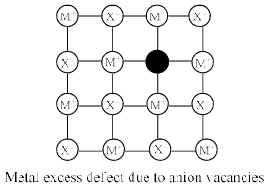(b) Metal Excess Defects due to Interstitial Cations : Another way in which metal excess defects may occur is, if an extra positive ion is present in an interstitial site. Electrical neutrality is maintained by the presence of an electron in the interstitial site. These t ypes of defects are exhibited by the crystals which are likely to exhibit ‘Frenkel’ defects. For example, when ZnO is heated, it loses oxygen reversibly. The excess metal is accommodated in interstitial sites, with electrons trapped in the neighbourhood. The yellow colour and the electrical conductivity of the non-stoichiometric ZnO is due to these trapped electrons.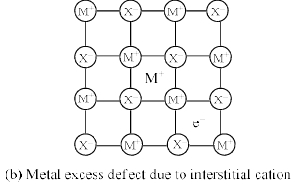(c) Metal Deficiency due to cations vacancies : The non-stoichiometric compounds may have metal deficiency due to the absence of a metal ion from its lattice site. The charge is balanced by an adjacent ion having higher positive charge. This type of defects are generally shown b y compounds of transition metals. It ma y be ment ioned here that metal excess compounds and metal defiant compounds both act as semi-conductors. Metal excess compounds conduct electricity through normal electron conduction mechanism and are therefore n-type semiconductors. Metal deficient compounds conduct electricity through positive hole conduction mechanism and are therefore p-type semiconductors.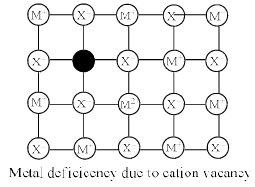(iii) Point Defects due to the Presence of Foreign Atoms (Impurity defect) : Foreign atoms can occupy interstitial sites in a crystal example non-stoichiometric cuprous oxide (Cu2O) can be prepared in laboratory. In this oxide copper to oxygen ratio is slightly less than 2:1. This is due to the reason that some of the positions which were to be occupied by Cu+ ions are vacant whereas some positions are occupied b y Cu2+ ions. Defects in the ionic solids may be introduced by adding impurity ions. If the impurity ions have different valence state than that of the host ions, vacancies are created. For example addition of SrCl2 to NaCl yields solids solution where the divalent cation occupies Na+ sites and produces cat ion vacancies equal to the number of the divalent ions occupying substitution sites.
Similarly, AgCl cr ystals can be doped with CdCl2 to produce impurity defects in a likewise manner.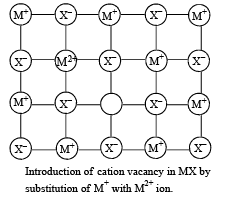Designation of Planes : The orientation of a lattice plane can be described by considering the intercepts of the plane on the three basis vectors of the lattice. According to Hauy, it is possible to choose the unit lengths a, b and c along the three basis vectors such that the ratio of each of the intercepts h`, k` and l` to the corresponding unit length is either an integer or a ratio of two integers. This statement is known as the law of rational indices. The three ratios are known as the Weiss indices of the plane.

Illustration: The three plane have intercepts:

(i) a, b, ∞ (ii) 2a, b, ∞ (iii) ∞, b, ∞

The intercepts are simple multiples of unit lengths a, b, c of the basis vectors along the crystallographic axes which determine the crystal system.
Miller indices (hkl) of such planes are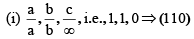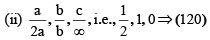(iii) Miller indices are (010)

Interplanar Distances for Cubic Systems : General formula for the interplanar spacing in the cubic system is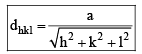Where hkl are Miller indices of the planes and a is length of the cube side.
If α, β and γ are the angles which this perpendicular makes with the three axes x, y and z respectively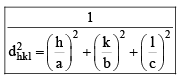Bragg’s Equation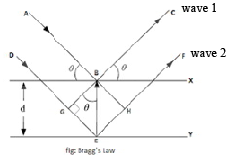In order to have wave 1 and wave 2 in phase, we must have 2d sin θ = nλ This is known as Bragg’s equation. The constant n gives the order of reflection and is equal to the number of wavelengths in the path difference between waves reflected by adjacent planes.

Offer running on EduRev: Apply code STAYHOME200 to get INR 200 off on our premium plan EduRev Infinity!

## Physical Chemistry

66 videos|94 docs|32 tests

,

,

,

,

,

,

,

,

,

,

,

,

,

,

,

,

,

,

,

,

,

;